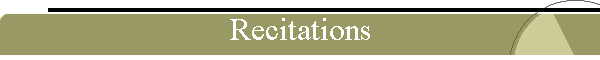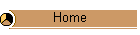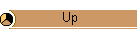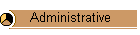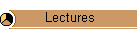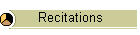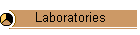Analytical Schedule of Recitation1st week Topics: Using computer mathematical notebooks (Studio Mathematics) Using computer algebra packages (Maxima, Scilab) Using an electrical and electronic circuit simulator (Qucs) Using an office package (Open Office) Using a graphics package (gnuplot)   Basic Points: Understand the basic capabilities and limitations of the software packages  Learn their operation  Simulate a number of examples that have been solved either analytically, or graphically, or both. Compare simulation and analytical results. Prepare a "Report"   Examples:   Applications: 2nd week Topics:  Analysis - Synthesis    Review of electric circuits: Special topics   Methodology:   Basic Points: Examples:  Representative examples:   Applications:  Study resistors and resistance   Study capacitors and capacitance   Study inductors and inductance   Study transformers and mutual inductance  Study voltage sources  Study current sources  Apply capacitors and inductors in practical circuits such as integrators and differentiators 3rd week Topics:   The electronic diode and applications Definition - Schematic symbol Typical voltage  current (v-i) characteristic of a diode  Mathematical description Physical and mathematical operation of a diode: Forward biased - Reversed biased Parameters of a diode: Dynamic resistance - Temperature effects Models of a diode: Ideal - Practical (Approximate) - Real Commercial diodes: Types - Data sheets - Specifications Measurements: Testing  Troubleshooting  Diode terminal identification Electronic circuits with diodes:  The Rectifying diode and applications The Zener diode and applications The Varactor diode and applications The Optical diode and applications The Current regulating diode and applications The Schottky diode and applications The PIN diode and applications The step recovery diode and applications The tunnel diode and applications The laser diode and applications Electronic systems with diodes: Power supply- block diagram - capacitor filter  capacitor inductor  capacitor inductor capacitor  capacitor resistor capacitor    Basic Points:  Diode characteristics    Analyze a diode circuit using analytical and graphical methods.  Synthesize a circuit using analytical and graphical methods.   Examples:  Representative examples:  Diodes_1a  Diodes_1b Applications: 4th week Topics:   The bipolar junction transistor (BJT) and applications Definition  schematic diagram Typical voltage current characteristics (v-i) of a transistor Physical and mathematical operation of a transistor Parameters of a transistor: Models of a transistor Commercial transistors: Types  Packaging - Datasheets  Specifications Measurements  Troubleshooting  Transistor terminal identification Electronic circuits with transistors: The transistor as an amplifier  The transistor as a switch   Methodology:   Basic Points:   Examples:  Representative examples:  BJT_1a BJT_1b Applications: 5th week Topics:   The field effect family of transistors and applications Definition  schematic diagram Typical voltage current characteristics (v-i) of a transistor Physical and mathematical operation of a transistor Parameters of a transistor Models of a transistor Commercial transistors: Types  Packaging - Datasheets  Specifications Measurements  Troubleshooting  Transistor terminal identification Biasing of a transistor  Operating point  Bias circuits Electronic circuits with transistors: The transistor as an amplifier  The transistor as a switch   Methodology:   Basic Points:   Examples:  Representative examples:FET_1aFET_1b   Applications: 6th week Topics:   The metal oxide semiconductor field effect transistor (MOSFET) and Applications Definition  schematic diagram Typical voltage current characteristics (v-i) of a transistor Physical and mathematical operation of a transistor Parameters of a transistor Models of a transistor Commercial transistors: Types  Packaging - Datasheets  Specifications Measurements  Troubleshooting  Transistor terminal identification Biasing of a transistor  Operating point  Bias circuits Electronic circuits with transistors: The transistor as an amplifier  The transistor as a switch     Operational amplifiers    Understand what operational amplifiers are   Learn about the ideal op amp   Learn some op amp circuits including inverting, non-inverting, summing, and difference amplifiers   Be able to use PSpice to analyze op amp circuits    Methodology:   Basic Points:   Examples:  Representative examples:OpAmp_1aOpAmp_1b     Applications: 7th week Topics:  Other electronic devices and applications a. The silicon controlled rectifier and applications b. The diac and applications c. The triac and applications d. The silicon controlled switch and applications e. The unijunction transistor and applications f. The programmable unijunction transistor and applications g. The IGBT   Methodology:   Basic Points:   Examples:  Representative examples:OpAmp_1aOpAmp_1b   Applications: 8th week Topics: Other electronic devices and applications a. The silicon controlled rectifier and applications b. The diac and applications c. The triac and applications d. The silicon controlled switch and applications e. The unijunction transistor and applications f. The programmable unijunction transistor and applications g. The IGBT   Methodology:   Basic Points: Understand what operational amplifiers are  Learn about the ideal op amp  Learn some op amp circuits including inverting, non-inverting, summing, and difference amplifiers  Be able to use a circuit simulator to analyze op amp circuits  Examples:  Representative examples:   Applications: 9th week Topics:  The operational amplifier and applications  a. Basic principles  b. Basic circuits  Operational Amplifiers  Ideal  Real  Voltage follower  Inverting  Summing  Difference   Methodology:   Basic Points:   Examples:  Representative examples:   Applications: 10th week Topics:   The operational amplifier and applications  a. Basic principles  b. Basic circuits  Operational Amplifiers  Ideal  Real  Voltage follower  Inverting  Summing  Difference   Methodology:   Basic Points: Examples:  Representative examples:   Applications:  Apply what is learnt to automobile ignition circuit and smoothing circuits 11th week Topics:  Part III: Electronic devices and basic systems 8. Small signal amplifiers a. One stage b. Two stages c. n-stages Laboratory session:   Methodology:   Basic Points: Examples: Representative examples:   Applications:  Apply what is learnt to phase-shifters and AC bridges 12th week Topics: 8. Small signal amplifiers a. One stage b. Two stages c. n-stages   Methodology:   Basic Points: Examples:  Representative examples:   Applications:  Apply what is learnt to capacitance multiplier and oscillators 13th week Topics:   Frequency response of the amplifiers  Introduction  Decibel  1. Low frequency response  2. Middle frequency response  3. High frequency response  4. Total amplifier frequency response  5. Multistage amplifier response   Methodology:   Basic Points: Examples:  Representative examples:   Applications:  Apply what is learnt to power measurement and electricity consumption cost 14th week Review:   Topics:   Frequency response of the amplifiers  Introduction  Decibel  1. Low frequency response  2. Middle frequency response  3. High frequency response  4. Total amplifier frequency response  5. Multistage amplifier response   Methodology:   Basic Points:   Examples:  Representative examples:   Applications:  Apply what is learnt to three-phase measurement and residential wiring 15th week Review:   Topics:   Large signal amplifiers  a. One stage with transformer  b. One stage without transformer  c. Push  pull  d. Complementary symmetry   Methodology:   Basic Points:   Examples:  Representative examples:   Applications: 16th week Final examination: